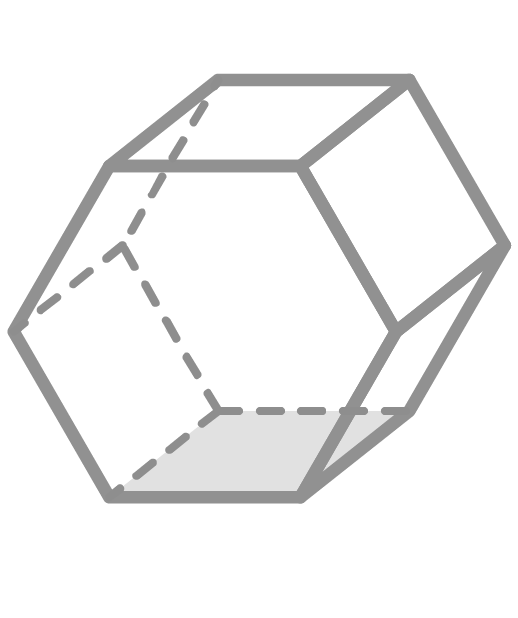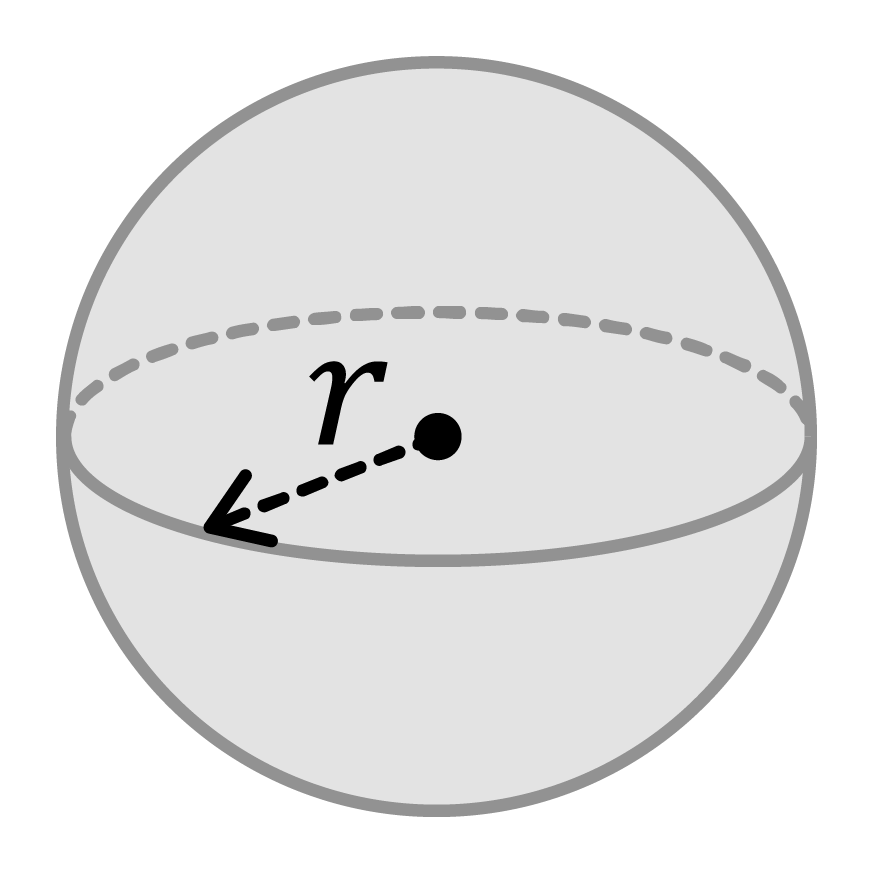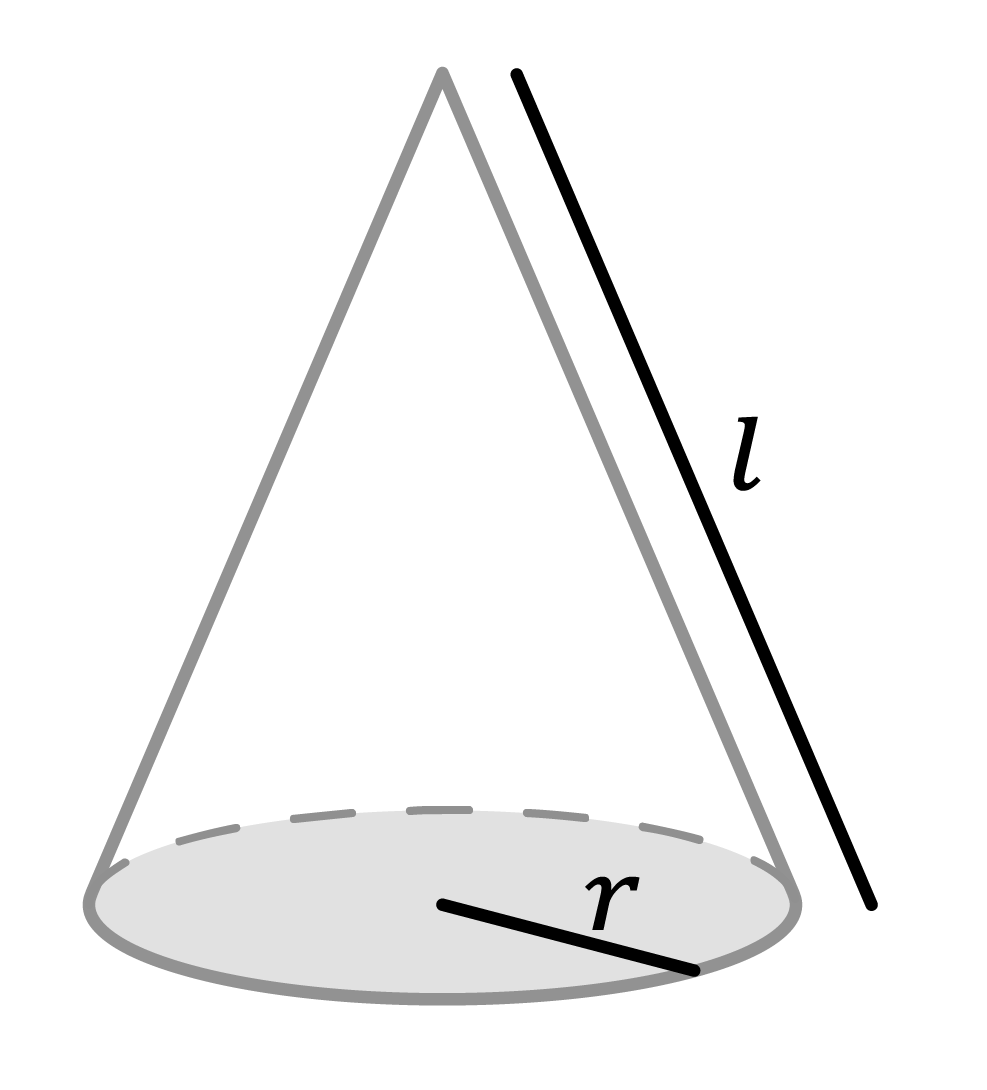Chapter OverviewMaths

Exam board

Pearson Edexcel

Number

Algebra

Graphs

Ratio proportion and rates of change

Shapes and area

Angles and geometry

Trigonometry

Probability

Statistics

Maths

# Surface area of 3D shapes: Nets, formulae0%

Summary

# Surface area of 3D shapes: Nets, formulae

## ​​In a nutshell

The surface area of a shape is the sum of the areas of its faces. The surface area of 3D shapes can either be calculated using nets or using given formulae.

## Surface area using nets

### Net

A net is a 3D shape that has been unfolded and laid out flat. Here are some examples of nets.

 SHAPE NET### Surface area

To find the surface area of a shape using nets, sketch the net and work out the area of the net. This can be done by working out the area of each individual shape in the net.

##### Example 1

What is the surface area of a cube with side lengths $8cm$?

First, sketch the net:

The net of a cube is six squares. In this example, each square will have side lengths $8cm$.

Then, find the area of the net:

The net is a compound shape consisting of six squares.

The area of one of these squares is given to be:

$8\times8=64cm^2$​​

The area of the net is therefore:

$6\times64=384cm^2$​​

The surface area of the cube is $\underline{384 \ cm^2}$.

## Surface area formulae

These are the formulae you need to know:

 SHAPE FORMULA DIAGRAM Sphere ​$S.A=4\pi r^2$​​Cylinder ​$S.A=2\pi r^2 +2\pi rh$​​Cone ​$\text{Curved surface area}=\pi rl$​​ ​$S.A=\pi rl +\pi r^2$​​##### Example 2

What is the surface area of a sphere with radius $2m$ to three significant figures?

Substitute $r=2$ into the formula:

\begin{aligned}S.A&=4\pi r^2\\&=4\pi (2)^2\\&=16\pi\\&=50.26548246...m^2\end{aligned}​​

The surface area is $\underline{50.3m^2\space\ (3\space s.f.)}$.

## Want to find out more? Check out these other lessons!

Nets and surface area

FAQs

• Question: Which shapes have a formula to work out their surface area?

Answer: There are given formulae to work out the surface area of a sphere, a cylinder and a cone.

• Question: How do you work out the surface area of a shape using nets?

Answer: First, sketch the net. Then, work out the area of the net by working out the area of each individual shape in the net.

• Question: What are the two ways of working out surface area?

Answer: The two ways of finding the surface area of a shape is to use a net or to use a formula.

Theory

Exercises